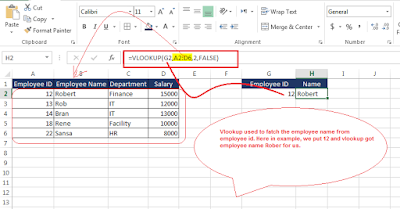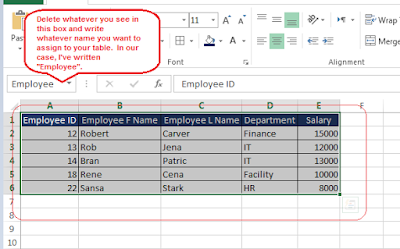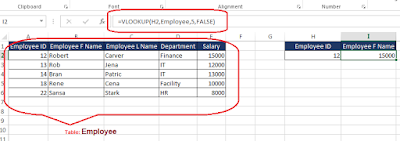5 Craziest Excel VLOOKUP Tricks You Must Know [EXPLAINED]We all know that Vlookup is one of the best feature or formula that Excel has provided in order to make reporting and preparing smart dashboards easier. Recently I came across some of the cool and craziest feature of VLookup which I would like to share with you guys and I’m sure you all love it. Let’s get started before you get bored with the introduction.VLOOKUP is one of the lookup or reference function used to find things in a Table or a range by row. Before I show the lookup tricks, I’m assuming you already know some of the basic feature of the same and just to revise the formula format see below,

To learn about other cool excel trick check out my other blog on Excel 7 Amazing MS Excel tricks which will take your reporting skill to the next level

There are four pieces of information that you will need in order to build the VLOOKUP syntax:
1. The value you want to look up, also called the lookup value.
2. The range where the lookup value is located. Remember that the lookup value should always be in the first column in the range for VLOOKUP to work correctly. For example, if your lookup value is in cell C2 then your range should start with C.
3. The column number in the range that contains the return value. For example, if you specify B2: D11 as the range, you should count B as the first column, C as the second, and so on.
4. Optionally, you can specify TRUE if you want an approximate match or FALSE if you want an exact match of the return value. If you don't specify anything, the default value will always be TRUE or approximate match.

Now put all of the above together as follows:
=VLOOKUP(lookup value, range containing the lookup value, the column number in the range containing the return value, optionally specify TRUE for approximate match or FALSE for an exact match).

1.Simple Use Of Lookup (Let’s start with simple one first to revise)

Consider a table with basic Employee details and we have been asked to find the employee name from employee ID.In this we were able to get employee name Robert using his Employee ID 12. It was the easy one, isn’t it? Let’s go little deeper.

2.Multi Value Vlookup Trick

What if we have been asked to fetch Employee salary and other employee details by using employee id?

Formula: =VLOOKUP(G2,A2:D6,{2,3,4},FALSE)We succeeded in getting Employee name, Department and Salary only with employee ID. Still easy right?

3.Table Naming Trick

This is one of the cool trick where you can name your table and use it with its name instead of range every time and also it’s good to use when you are using multiple table to generate dashboards.
Below are the steps for naming the table:

Select the table range which you want to use and go to left top corner to name it.We selected range A1:E6 and named this table "Employee", so that next time we can use this range with some meaningful word.

Now, how to use?
Let’s go back to previous example to find the salary from employee id.Formula applied on Column I2 and its =VLOOKUP (H2, Employee, 5, FALSE)

If you noticed, we used "Employee" instead of table range. Looks good right?

4. Customer Error Trick

Showing customer error in case of required record is missing in master table.
In some of the cases we need to show customer error for better and clear output. Please are the steps to achieve this.
Formula Used: =IFERROR (VLOOKUP (H3, Employee, 5, FALSE),"Not Found")
Image 4.1You can see that instead of shoing default meaning less error it is showing custom error "Not Found" for missing records. we don't have employee details for the employee ID 40.

Note: Naming table has been used instead of range, If you don’t recall then go back to trick 3.

5.Two Dimensional Lookup Trick

Under usual circumstances while using VLOOKUP we hardcode the column number from which Excel should get the result. Consider the following formula:

=VLOOKUP(G5,B2:C10,2)

“2” is actually column number that we mentioned. But we may have situations where our data is spread in different columns, and we need to get the right column first based on criteria and then we perform the lookup. This is called 2D lookup or two-dimensional or 2 criteria lookup. It goes with many names.

Consider below scenario where price needs to be selected based on product + size.Let’s understand the vlookup fomula:
=VLOOKUP(H8,A8:D12,MATCH(H9,\$A\$8:\$D\$8,0),FALSE)

Here, vlookup first check the value in column H8 and search the value in column mentioned in H9. In above example lookup searched for shirt price in column M.

This was cool, isn’t it?

Make sure to check below to avoid error while practising.

• Watch the Phantom Extra Space – Vlookups don’t work if the Lookup Value has unwanted extra spaces. Use the Trim function to get rid of any extra spaces in the cell and then apply  the VLookup. =TRIM(Cell Reference)
• Search Column is the First Column- The VLOOKUP formula only looks in the first column of the select data
• Not freezing the Table Array Range – The VLOOKUP freezes the range automatically if you are applying it to another workbook, but if working in the same sheet, you have to do it manually using the F4 Key
• Leaving the Range Lookup Blank – By default excel applies an approximate match (TRUE), make sure if you applying an exact match you specify FALSE as an input
• =VLOOKUP(lookup_value,table_array,col_index_num) – this will automatically pick up TRUE or approximate match
• =VLOOKUP(lookup_value,table_array,col_index_num,0) – this also means FALSE
• =VLOOKUP(lookup_value,table_array,col_index_num,) – if you dont write anything after the comma, this also means FALSE

To learn about other cool excel trick check out my other blog on Excel 7 Amazing MS Excel tricks which will take your reporting skill to the next level

Excel experts may find these tricks easy but it's good and makes difference while preparing various reports and dashboards. These tricks are easy to understand and I believe every individual dealing with excel file should know. I hope you learned something new in this article.

If you liked this article then don't forget to like us on facebook, widget is there on right-top corner of this article. :) If you have any question of feedback, feel free to rite in comment section of this article.

This post first appeared on Buzz Analysis, please read the originial post: here

Share the post

5 Craziest Excel VLOOKUP Tricks You Must Know [EXPLAINED]

×

Subscribe to Buzz Analysis

Get updates delivered right to your inbox!

×